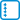## Triangulations of an n-gon

How many distinct triangulations of a regular $$n$$-gon with labeled vertices exist?

• #### Solution

Denote the vertices cyclically $$A_1,A_2,\ldots,A_n$$ and consider in the triangulation the triangle containing the edge $$A_1A_2$$. Let its third vertex be $$A_k$$, $$k\in\{3,\ldots,n\}$$. The number of possible triangulations containing the triangle $$A_1,A_2,A_k$$ is then equal to $$t_{k-1}t_{n-(k-2)}$$ Here $$t_{k-1}$$ is the number of triangulations of the $$(k-1)$$-gon $$A_2,A_3,\ldots,A_k$$ and $$t_{n-(k-2)}$$ is the number of triangulations of the $$n-(k-2)$$-gon $$A_1,A_k,\ldots,A_n$$.

For the case when the triangle $$A_1,A_2,A_k$$ has two edges incident with the boundary, we set $$t_2=1$$. We obtain the recurrence:

$$\displaystyle t_n=\sum_{k=3}^n t_{k-1}t_{n-(k-2)} = \sum_{k=2}^{n-1} t_k t_{n+1-k}.$$

For better clarity, we rewrite without the sum

$$t_n=t_2t_{n-1}+t_3t_{n-2}+\ldots+t_{n-2}t_3+t_{n-1}t_2$$

or

$$t_{n+2}=t_2t_{n+1}+t_3t_n+\ldots+t_nt_3+t_{n+1}t_2$$

After the substitution $$b_n=t_{n+2}$$ we obtain the recurrence:

$$b_n=b_0b_{n-1}+b_1b_{n-2}+\ldots+b_{n-2}b_1+b_{n-1}b_0$$

It can be seen that we get the same recurrence as for the Catalan numbers $$b_n$$ including the same initial conditions, i.e. $$b_0=t_2=1$$.

The given $$n$$-gon can be triangulated in $$\frac{1}{n-1}\binom{2n-4}{n-2}$$ distinct ways.